# Viacheslav Borovitskiy (Slava)# Gaussian Processes

Gaussian process $f$ — random function with jointly Gaussian marginals.

Characterized by

• a mean function $m(x) = \E(f(x))$,
• a kernel (covariance) function $k(x, x') = \Cov(f(x), f(x'))$.

Notation: $f \~ \f{GP}(m, k)$.

The kernel $k$ must be positive (semi-)definite.

# Gaussian Process Regression

Takes

• data $(x_1, y_1), .., (x_n, y_n) \in X \x \R$,
• and a prior Gaussian Process $\f{GP}(m, k)$

giving the posterior (conditional) Gaussian process $\f{GP}(\hat{m}, \hat{k})$.

The functions $\hat{m}$ and $\hat{k}$ may be explicitly expressed in terms of $m$ and $k$.

# Consider a Simple Dynamical System: a Pendulum

$$\htmlData{fragment-index=0,class=fragment}{ x_0 } \qquad \htmlData{fragment-index=1,class=fragment}{ x_1 = x_0 + f(x_0)\Delta t } \qquad \htmlData{fragment-index=2,class=fragment}{ x_2 = x_1 + f(x_1)\Delta t } \qquad \htmlData{fragment-index=3,class=fragment}{ .. }$$

# Consider a Simple Dynamical System: a Pendulum

$$x_0 \qquad x_1 = x_0 + f(x_0)\Delta t \qquad x_2 = x_1 + f(x_1)\Delta t \qquad ..$$

# Consider a Simple Dynamical System: a Pendulum

$$f: \R \x \R \to \R \x \R$$

assume $f$ unknown, model $f$ as a GP.

$$x_0 \qquad x_1 = x_0 + f(x_0)\Delta t \qquad x_2 = x_1 + f(x_1)\Delta t \qquad ..$$

# Pendulum Dynamics: Phase Portrait

Phase portrait is periodic!

# Pendulum Dynamics: Phase Portrait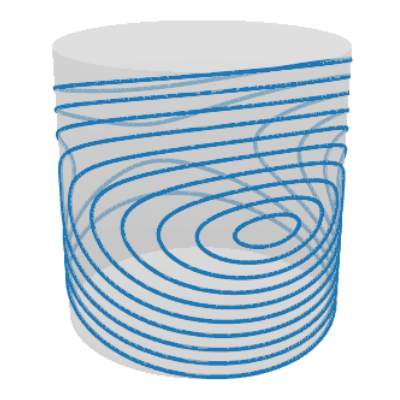This means that the state space is actually a cylinder!

# Pendulum Dynamics: Phase PortraitPhase portrait is periodic!I.e. the state space is actually a cylinder!

# Need priors on non-Euclidean domains!

Be able to:     evaluate $k(x, x')$,     differentiate it,      sample $\mathrm{GP}(0, k)$.

# Non-Euclidean Domains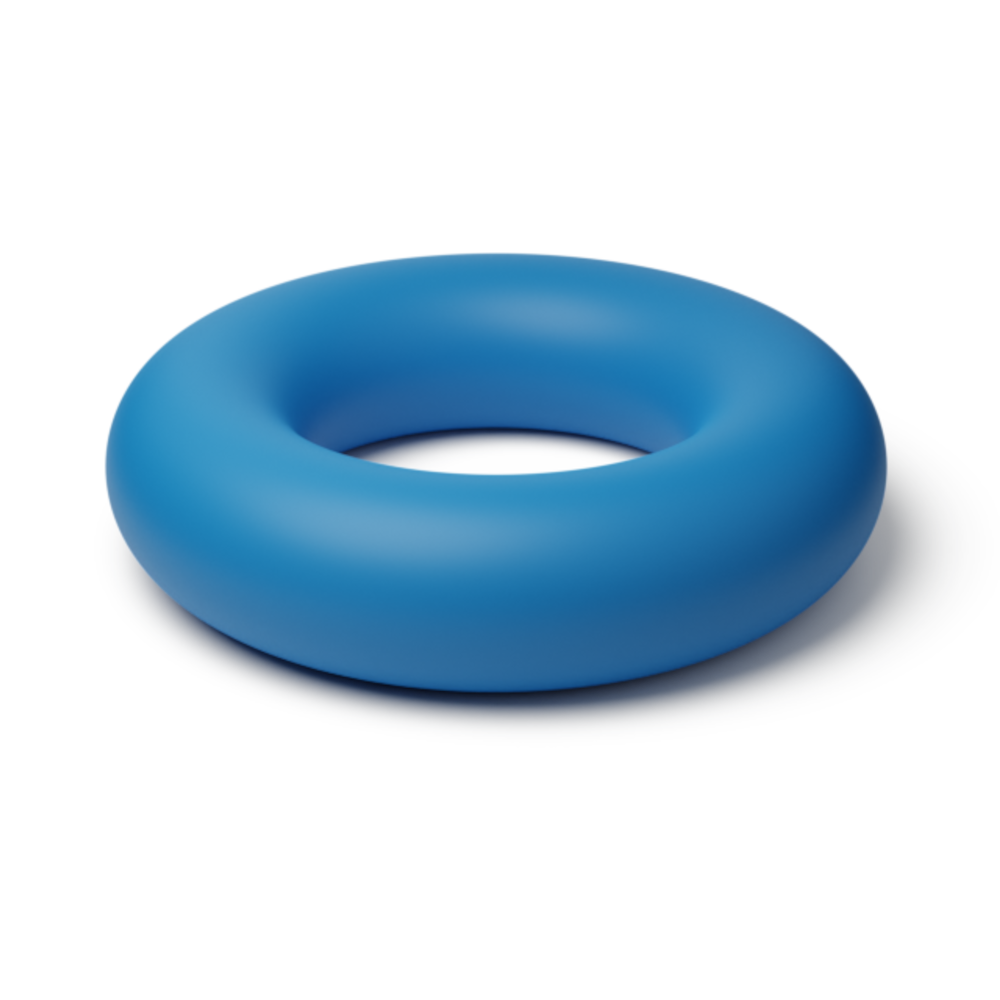### Manifolds e.g. in physics (robotics)### Graphs e.g. for road networks.# Matérn Gaussian Processes

$$\htmlData{class=fragment fade-out,fragment-index=6}{ \footnotesize \mathclap{ k_{\nu, \kappa, \sigma^2}(x,x') = \sigma^2 \frac{2^{1-\nu}}{\Gamma(\nu)} \del{\sqrt{2\nu} \frac{\abs{x-x'}}{\kappa}}^\nu K_\nu \del{\sqrt{2\nu} \frac{\abs{x-x'}}{\kappa}} } } \htmlData{class=fragment d-print-none,fragment-index=6}{ \footnotesize \mathclap{ k_{\infty, \kappa, \sigma^2}(x,x') = \sigma^2 \exp\del{-\frac{\abs{x-x'}^2}{2\kappa^2}} } }$$ $\sigma^2$: variance $\kappa$: length scale $\nu$: smoothness
$\nu\to\infty$: RBF kernel (Gaussian, Heat, Diffusion)$\nu = 1/2$$\nu = 3/2$$\nu = 5/2$$\nu = \infty$

# Distance-based Approach

$$k_{\infty, \kappa, \sigma^2}(x,x') = \sigma^2\exp\del{-\frac{|x - x'|^2}{2\kappa^2}}$$

$$k_{\infty, \kappa, \sigma^2}^{(d)}(x,x') = \sigma^2\exp\del{-\frac{d(x,x')^2}{2\kappa^2}}$$

It is geometry-aware, but...

For manifolds. Not well-defined unless the manifold is isometric to a Euclidean space.
(Feragen et al. 2015)

For graphs. Not well-defined unless nodes can be isometrically embedded into a Hilbert space.
(Schonberg, 1930s)

For spaces of graphs. What is a space of graphs?!

# Another Approach: Stochastic Partial Differential Equations

$$\htmlData{class=fragment,fragment-index=0}{ \underset{\t{Matérn}}{\undergroup{\del{\frac{2\nu}{\kappa^2} - \Delta}^{\frac{\nu}{2}+\frac{d}{4}} f = \c{W}}} }$$ $\Delta$: Laplacian $\c{W}$: Gaussian white noise $d$: dimension

# Making It Explicit: Compact Manifolds

Examples: $\bb{S}_d$, $\bb{T}^d$

# Solution for Compact Riemannian Manifolds

The solution is a Gaussian process with kernel $$\htmlData{fragment-index=2,class=fragment}{ k_{\nu, \kappa, \sigma^2}(x,x') = \frac{\sigma^2}{C_{\nu, \kappa}} \sum_{n=0}^\infty \Phi_{\nu, \kappa}(\lambda_n) f_n(x) f_n(x') }$$

## $\lambda_n, f_n$: eigenvalues and eigenfunctions of the Laplace–Beltrami operator

$$\htmlData{fragment-index=4,class=fragment}{ \Phi_{\nu, \kappa}(\lambda) = \begin{cases} \htmlData{fragment-index=5,class=fragment}{ \del{\frac{2\nu}{\kappa^2} - \lambda}^{-\nu-\frac{d}{2}} } & \htmlData{fragment-index=5,class=fragment}{ \nu < \infty \text{ --- Matérn} } \\ \htmlData{fragment-index=6,class=fragment}{ e^{-\frac{\kappa^2}{2} \lambda} } & \htmlData{fragment-index=6,class=fragment}{ \nu = \infty \text{ --- Heat (RBF)} } \end{cases} }$$

# How to get $\lambda_n, f_n$?

Discretize the Problem

— works for general manifolds of very low dimension,

— see «Matérn Gaussian processes on Riemannian manifolds», NeurIPS 2020.

Use Algebraic Structure

— works for homogenous spaces (e.g. $\mathbb{S}_d$ or $\mathrm{SO}(n)$) of higher dimension,

— see «Stationary Kernels and Gaussian Processes on Lie Groups and their Homogeneous Spaces I», under review at JMLR, 2023.

# Examples: The Values of $k_{1/2}(\htmlStyle{color:rgb(255, 19, 0)!important}{\bullet},\.)$ on Compact Riemannian ManifoldsCircle
(Lie group)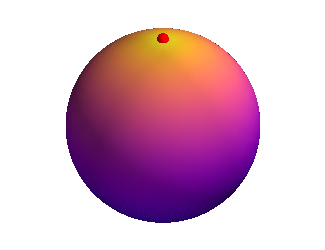Sphere
(homogeneous space)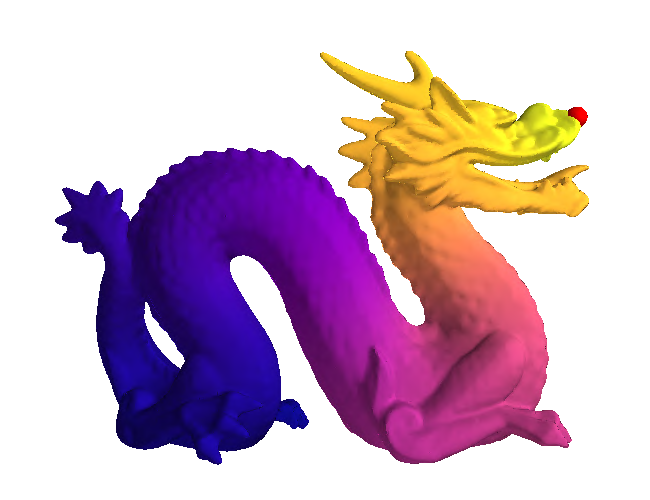Dragon
(general manifold)

# Examples: Samples from $\mathrm{GP}(0, k_{\infty})$ on Compact Homgeneous Spaces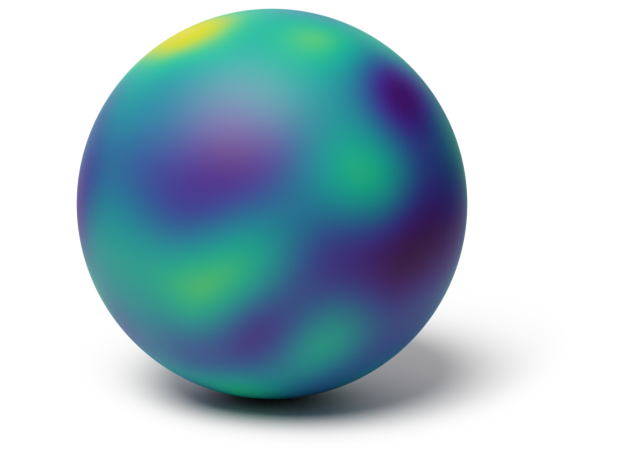Sphere $\mathbb{S}_2$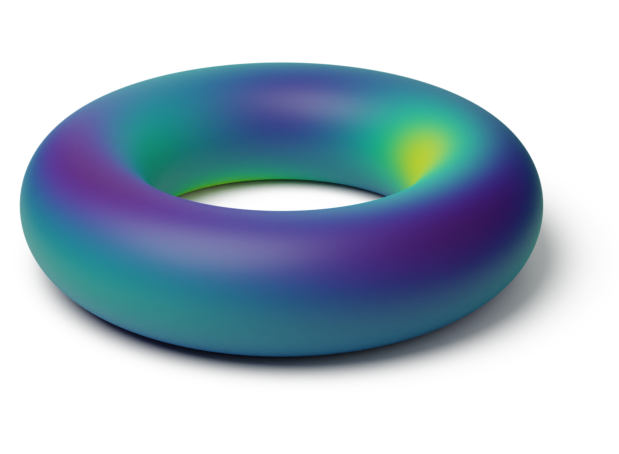Torus $\mathbb{T}^2 = \bb{S}_1 \x \bb{S}_1$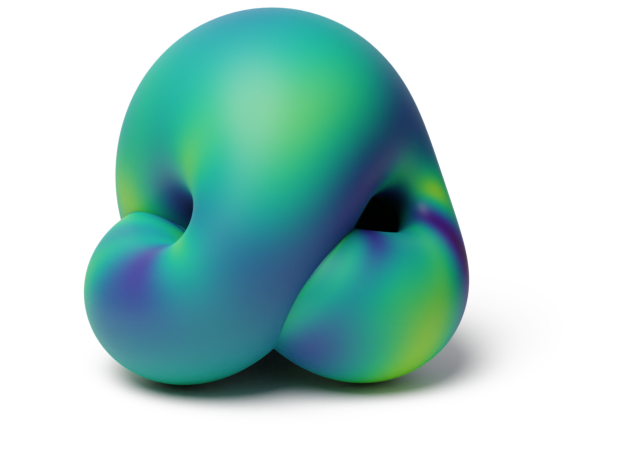Projective plane $\mathrm{RP}^2$

# Making It Explicit: Non-compact Manifolds

Examples: $\bb{H}_d$, $\mathrm{SPD}(d)$

# The Basic Idea

For symmetric spaces like $\bb{H}_d$,  $\mathrm{SPD}(d)$ harmonic analysis generalizes well.

This allows to generalize random Fourier features to this setting.

— a few technical challenges arise though.

— see «Stationary Kernels and Gaussian Processes on Lie Groups and their Homogeneous Spaces II»—under review at JMLR, 2023.

# Examples: The Values of $k_{\infty}(\htmlStyle{color:rgb(0, 0, 0)!important}{\bullet},\.)$ on Non-compact Manifolds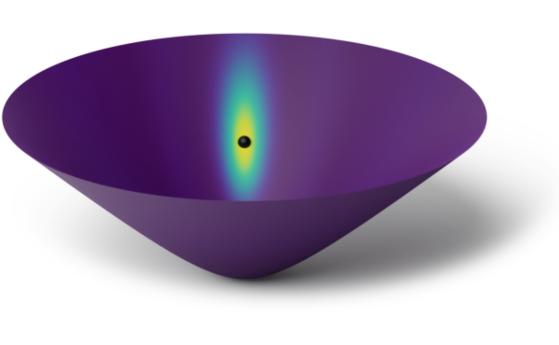Hyperbolic space $\bb{H}_2$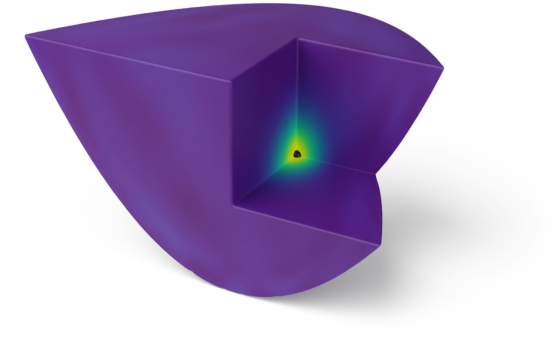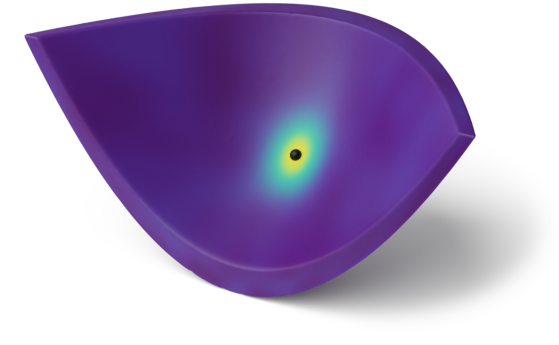Space of positive definite matrices $\f{SPD}(2)$

# Examples: Samples from $\mathrm{GP}(0, k_{\infty})$ on Non-compact Homgeneous Spaces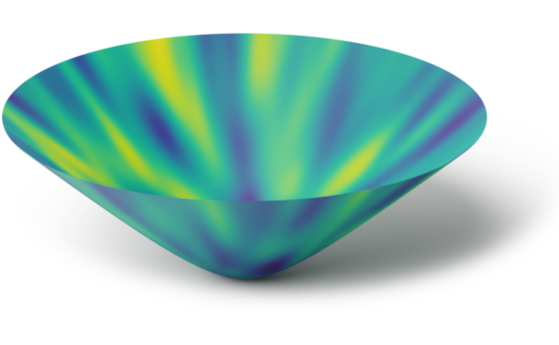Hyperbolic space $\bb{H}_2$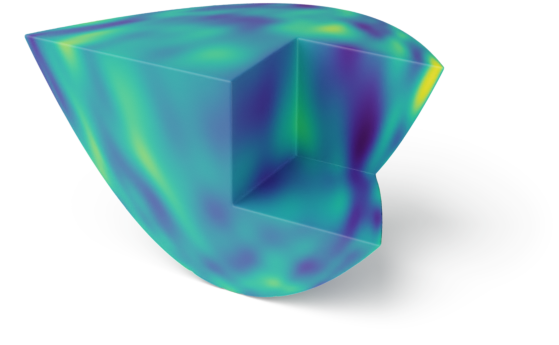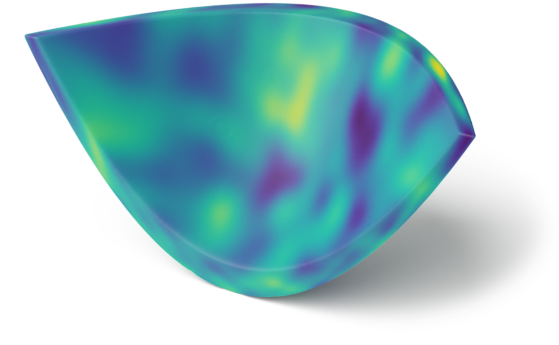Space of positive definite matrices $\f{SPD}(2)$

# Application: Bayesian Optimization in Robotics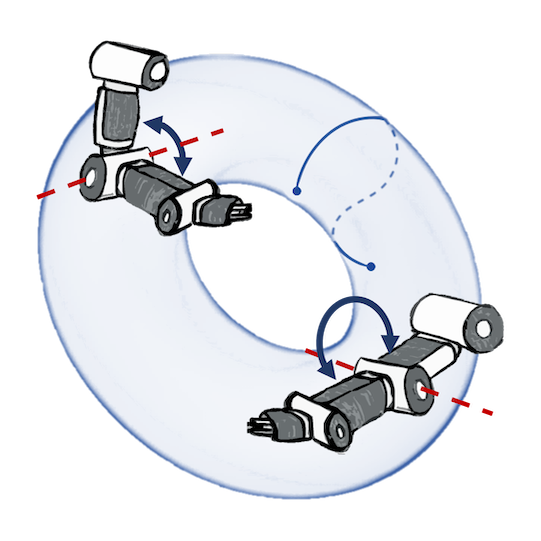### Joint postures: $\mathbb{T}^d$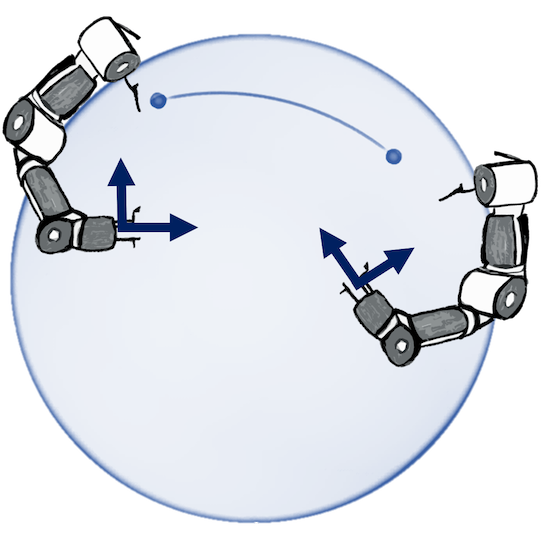### Rotations: $\mathbb{S}_3$, $\operatorname{SO}(3)$# Application: Wind Speed Modeling (Vector Fields on Manifolds)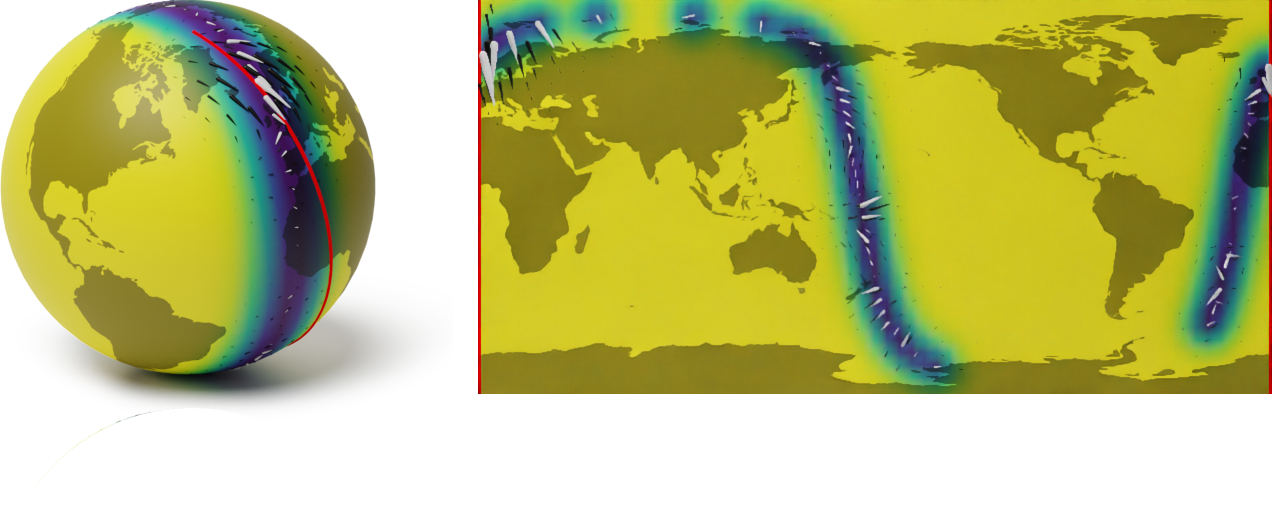Geometry-aware vs Euclidean

# Matérn Kernels on Weighted Undirected Graphs

SPDE turns into a stochastic linear system. The solution has kernel $$\htmlData{fragment-index=2,class=fragment}{ k_{\nu, \kappa, \sigma^2}(i, j) = \frac{\sigma^2}{C_{\nu, \kappa}} \sum_{n=0}^{\abs{V}-1} \Phi_{\nu, \kappa}(\lambda_n) \mathbf{f_n}(i)\mathbf{f_n}(j) }$$

## $\lambda_n, \mathbf{f_n}$: eigenvalues and eigenvectors of the Laplacian matrix

$$\htmlData{fragment-index=4,class=fragment}{ \Phi_{\nu, \kappa}(\lambda) = \begin{cases} \htmlData{fragment-index=5,class=fragment}{ \del{\frac{2\nu}{\kappa^2} - \lambda}^{-\nu} } & \htmlData{fragment-index=5,class=fragment}{ \nu < \infty \text{ --- Matérn} } \\ \htmlData{fragment-index=6,class=fragment}{ e^{-\frac{\kappa^2}{2} \lambda} } & \htmlData{fragment-index=6,class=fragment}{ \nu = \infty \text{ --- Heat (RBF)} } \end{cases} }$$

# Traffic Speed Extrapolation on a Road Network### (a) Prediction# Spaces of Graphs

Consider the set of all unweighted graphs with $n$ nodes. It is finite!
How to give it a geometric structure? Make it into a space?

### Graphs define geometry of discrete sets. We make it into a node set of a graph!# Spaces of Graphs

Beyond functions of actual graphs $f\big(\smash{\includegraphics[height=2.5em,width=1.0em]{figures/gg2.svg}}\big)$, it is useful to consider functions of equivalence classes of graphs: $f\big(\big\{\smash{\includegraphics[height=2.5em,width=1.0em]{figures/gg2.svg}}, \smash{\includegraphics[height=2.5em,width=1.0em]{figures/gg3.svg}}, \smash{\includegraphics[height=2.5em,width=1.0em]{figures/gg4.svg}}\big\}\big)$.# Spaces of Graphs### Space of graphs with $n = 3$ nodes as the $3$-dimensional cube# Spaces of Graphs### Space of graphs with $n = 3$ nodes as the $3$-dimensional cube# Application: Drug Design# Implementation# Together with# AlexanderTerenin# PeterMostowsky# IskanderAzangulov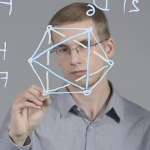# AndreiSmolensky# Vignesh RamSomnath# NoémieJaquier# MichaelHutchinson# SoTakao# Thank you!

Slides:
viacheslav.borovitskiy@gmail.com                       https://vab.im